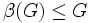# Left-transitively homomorph-containing not implies subhomomorph-containing

This article gives the statement and possibly, proof, of a non-implication relation between two subgroup properties. That is, it states that every subgroup satisfying the first subgroup property (i.e., left-transitively homomorph-containing subgroup) need not satisfy the second subgroup property (i.e., subhomomorph-containing subgroup)
View a complete list of subgroup property non-implications | View a complete list of subgroup property implications
Get more facts about left-transitively homomorph-containing subgroup|Get more facts about subhomomorph-containing subgroup
EXPLORE EXAMPLES YOURSELF: View examples of subgroups satisfying property left-transitively homomorph-containing subgroup but not subhomomorph-containing subgroup|View examples of subgroups satisfying property left-transitively homomorph-containing subgroup and subhomomorph-containing subgroup

## Proof

Let$G$ be the direct product:$G := A_5 \times C_2$

where$A_5$ is the alternating group of degree five and$C_2$ is the cyclic group of order two. Let$H$ be the first direct factor.

Then:

• For any group$K$ containing$G$ as a homomorph-containing subgroup,$K$ also contains$H$ as a homomorph-containing subgroup: For any homomorphism$\alpha:H \to K$, we can extend it to a homomorphism$\beta:G \to K$, since$H$ is a direct factor of$G$. Since$G$ is homomorph-containing in$K$,$\beta(G) \le G$, so$\alpha(H) \le G$. Now, since$H$ is homomorph-containing in$G$,$\alpha(H)$ is contained in$H$.
•$H$ is not a subhomomorph-containing subgroup of$G$:$H$ has cyclic subgroups of order two, that are isomorphic to cyclic subgroups of order two in$G$ and outside$H$.